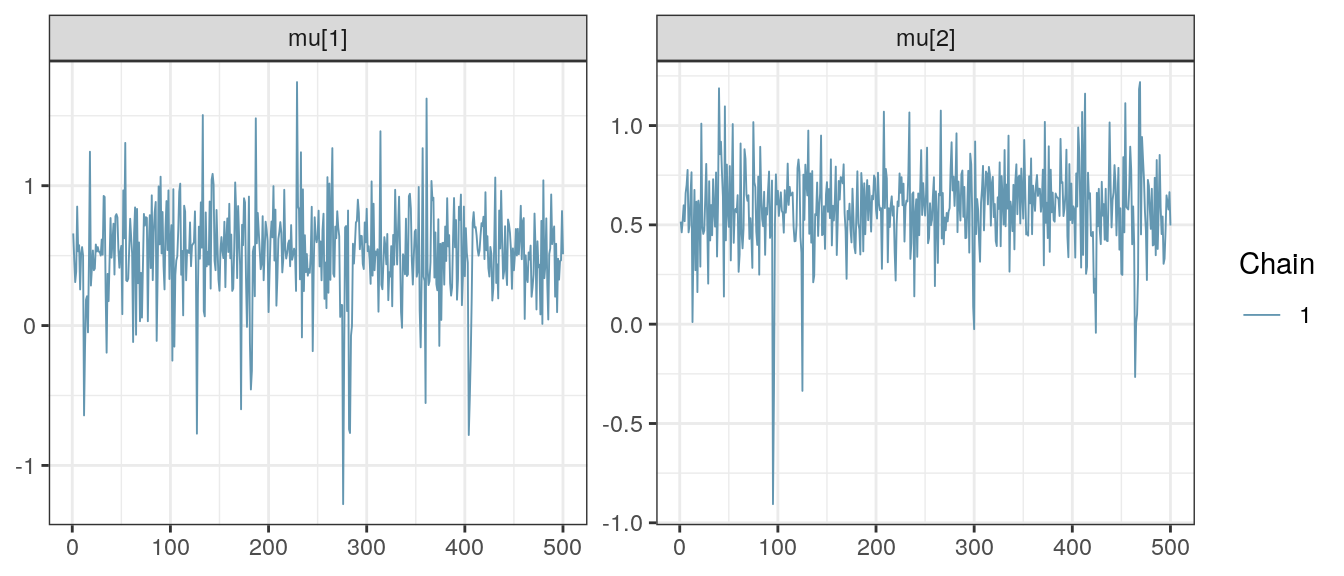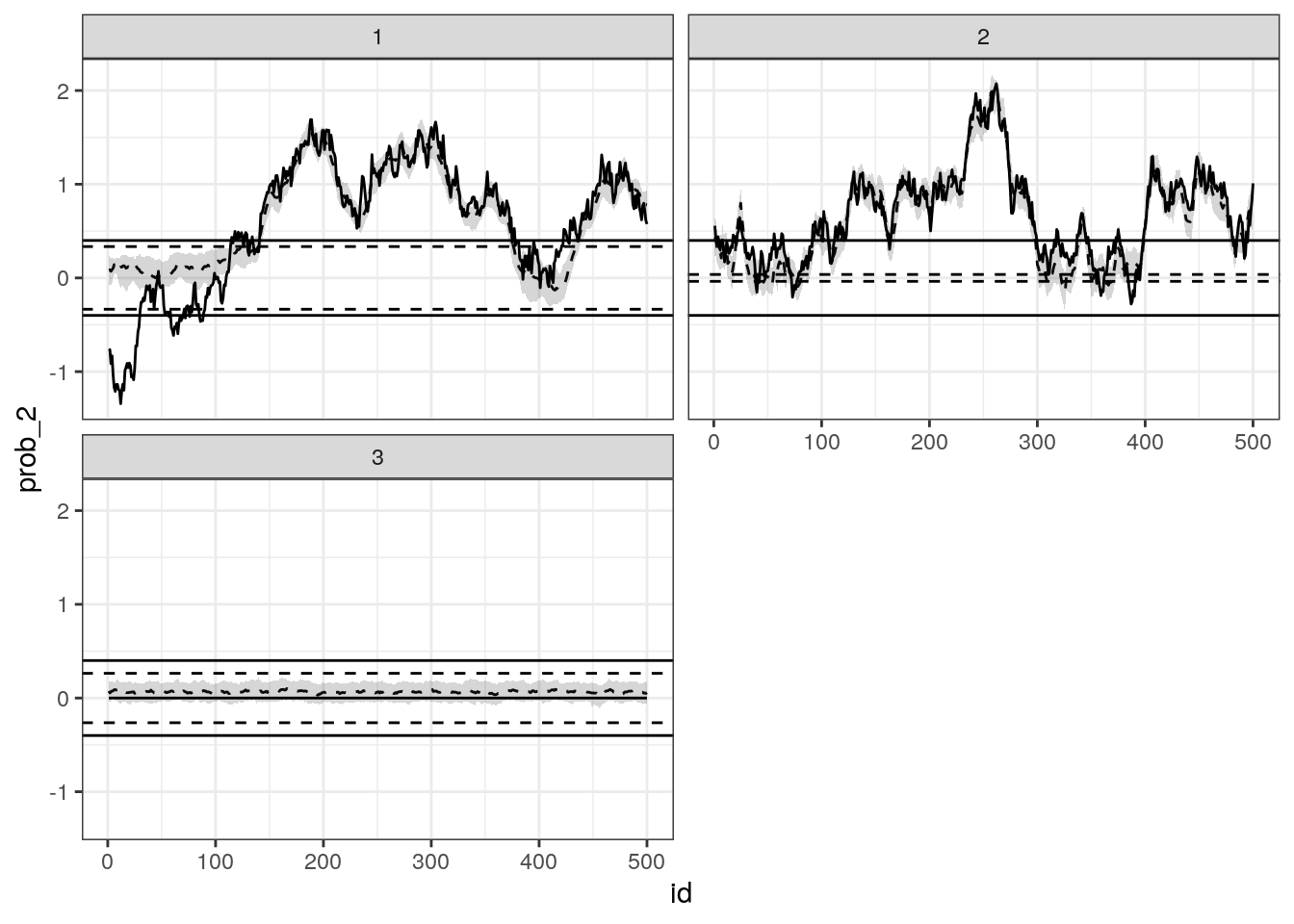The goal of bltm is to fit Bayesian Latent Threshold Models using R. The model in the AR(1) form is defined by these equations:

\begin{aligned} y_{it} &= \sum_{j=1}^J x_{ijt} b_{ijt} + \varepsilon_{it} \\ b_{ijt} &= \beta_{ijt} \,\mathbb I(|\beta_{ijt}| \geq d_{ij}) \\ \beta_{ij,t+1} &= \mu_{ij} + \phi_{ij}(\beta_{ijt}-\mu_{ij}) + \eta_{ijt} \end{aligned}

for $$i \in 1,\dots, I$$, $$j \in 1,\dots, J$$ and $$t \in 1,\dots, T$$. These models can be fit separatedly for each $$i$$. The example below fits the model to one single series ($$I=1$$).

library(tidyverse)
#> ── Attaching packages ───────────────────────────────────────────────────────────────────── tidyverse 1.2.1 ──
#> ✔ ggplot2 3.2.0          ✔ purrr   0.3.2
#> ✔ tibble  2.1.3          ✔ dplyr   0.8.1
#> ✔ tidyr   0.8.3.9000     ✔ stringr 1.4.0
#> ✔ readr   1.3.1          ✔ forcats 0.4.0
#> ── Conflicts ──────────────────────────────────────────────────────────────────────── tidyverse_conflicts() ──
#> Loading bltm

## Simulated Example

set.seed(103)

d_sim <- ltm_sim(
ni = 5, ns = 500, nk = 2,
alpha = 0,
vmu = matrix(c(.5,.5), nrow = 2),
mPhi = diag(2) * c(.99, .99),
mSigs = c(.1,.1),
dsig = .15,
vd = matrix(c(.4,.4), nrow = 2)
)

binder <- array(runif(500)-.5, c(5, 500, 1))
d_sim$mx <- abind::abind(d_sim$mx, binder, along = 3)
d_sim$mb <- cbind(d_sim$mb, 0)
result <- ltm_mcmc(d_sim$mx, d_sim$vy, burnin = 100, iter = 500)
# readr::write_rds(result, "data-raw/result.rds", compress = "xz")
Iteration:     1 /   600 [  0%]  (Warmup)
Iteration:    60 /   600 [ 10%]  (Warmup)
Iteration:   120 /   600 [ 20%]  (Sampling)
Iteration:   180 /   600 [ 30%]  (Sampling)
Iteration:   240 /   600 [ 40%]  (Sampling)
Iteration:   300 /   600 [ 50%]  (Sampling)
Iteration:   360 /   600 [ 60%]  (Sampling)
Iteration:   420 /   600 [ 70%]  (Sampling)
Iteration:   480 /   600 [ 80%]  (Sampling)
Iteration:   540 /   600 [ 90%]  (Sampling)
Iteration:   600 /   600 [ 100%]  (Sampling)

## Results

Results after 100 burnin and 500 iterations.

result <- read_rds("data-raw/result.rds")

vars_to_analyse <- !str_detect(colnames(result), "beta\$") summary_table <- result[,vars_to_analyse] %>% as.data.frame() %>% tibble::rowid_to_column() %>% tidyr::gather(key, val, -rowid) %>% dplyr::group_by(key) %>% dplyr::summarise( median = median(val), sd = sd(val), q025 = quantile(val, 0.025), q975 = quantile(val, 0.975) ) knitr::kable(summary_table) key median sd q025 q975 alpha 0.0076648 0.1781915 -0.3405058 0.3593770 alpha -0.0054158 0.1830903 -0.3785451 0.3289486 alpha -0.0047880 0.1834158 -0.3854517 0.3297607 alpha -0.0163057 0.1746813 -0.3578320 0.2923298 alpha -0.0016525 0.1801260 -0.3304960 0.3382569 d 0.3293945 0.0496160 0.2324488 0.4076028 d 0.0150496 0.0559342 0.0150496 0.1553439 d 0.2701171 0.0146839 0.2296899 0.2701171 mu 0.5296798 0.3416395 -0.2345459 1.0597430 mu 0.5947912 0.2164022 0.1596099 1.0128513 mu 0.0665906 0.0173249 0.0260728 0.0945483 phi 0.9904074 0.0050235 0.9784768 0.9977583 phi 0.9713490 0.0113390 0.9458727 0.9921012 phi 0.6437641 0.1325909 0.3581005 0.8528770 sig_eta 0.0731782 0.0083558 0.0561148 0.0888805 sig_eta 0.1222165 0.0138444 0.0921127 0.1494326 sig_eta 0.0623744 0.0100680 0.0486990 0.0867437 sig 0.1760752 0.0045631 0.1677408 0.1878592 ### MCMC Chains bayesplot::mcmc_trace(result, regex_pars = "mu\\[") + theme_bw()### Estimated Betas # check this function inside demo/ folder source("demo/plot_betas.R") plot_betas(result, 1:3, real_values = d_sim) + facet_wrap(~factor(p), ncol = 2) + theme_bw()## Model details ### Sampling from $$\alpha_i^{*}$$ \[\bar y_i = \frac 1 T \sum_{t=1} y_{it}$

$\mu^{*}_{\alpha} = \frac{a^*T + \mu_{\alpha}^0 s_{\alpha}^0}{T + s_{\alpha}^0},$

where

$a^*_i=\frac{1}{K}\sum_{t=1}^{T}\sum_{k=1}^{K}x_{itk}\beta_{tk}$

Generate using

$\boldsymbol\alpha^{*} \sim\mathcal N(\mu_{\alpha}^{*}, \sigma^{*2}),$

where $$\sigma^{*2}$$ is the posterior sample of $$\sigma$$

### Sampling from $$\boldsymbol\beta$$

The difference between the paper and the code is that it checks if some of the $$\boldsymbol\beta$$ should be replaced by zero. Actually, it is not a difference; the appendix is just a little obscure in this passage.

First, we calculate $$M_t$$ and $$m_t$$ (depending on $$t$$), then we generate candidates for $$\beta_t$$ and their log-densities using the normal distribution.

We then recalculate $$M_t$$ and $$m_t$$, replacing some of $$x_t$$s entries by zero, depending on a condition based on the threshold and the values of $$\boldsymbol\beta$$. Finally, we obtain the log-density of the previously calculated $$m_t$$ using these new parameters. This is the MH step where we draw a candidate from a (auxiliary) posterior distribution of non-threshold model. When we decide to accept the candidate, we compute the posterior distribution of the auxiliary posterior distribution and the true posterior distribution (of the threshold model). For the latter, we need to replace some of $$x_t$$’s entries by zero depending on a condition based on the threshold and the betas.

It’s important to note that if there aren’t any $$\beta$$ to replace by zero, then we should accept the new $$\boldsymbol\beta$$ with probability one, because the posterior has an analytic formula.